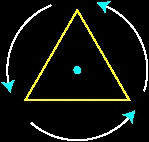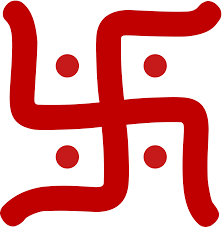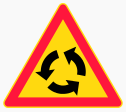# Rotational Symmetry

The rotational symmetry of a shape explains that when an object is rotated on its own axis, the shape of the object looks the same. Many geometrical shapes appear to be symmetrical when they are rotated 180 degrees or with some angles, clockwise or anticlockwise. Some of the examples are square, circle, hexagon, etc. A scalene triangle does not have symmetry if rotated since the shape is asymmetrical. By the word symmetry, we know it is a combination of two words ‘sync+metry’. Hence, there should be at least two identical order to have symmetry.

You may have often heard of the term ‘symmetry’ in day-to-day life. It is a balanced and proportionate similarity found in two halves of an object, that is, one-half is the mirror image of the other half. And a shape that is not symmetrical is referred to as asymmetrical. Symmetry is found all around us, in nature, in architecture and in art. It may be explored when you flip, slide or turn an object. There may be different types of symmetry:

• Reflection
• Translational
• Rotational

## What is Rotational Symmetry?

If a figure is rotated around a centre point and it still appears exactly as it did before the rotation, it is said to have rotational symmetry. A number of shapes like squares, circles, regular hexagon, etc. have rotational symmetry.

There are many shapes you will see in geometry which are symmetrical rotationally, such as:

• Equilateral triangles
• Squares
• Rectangles
• Circles
• Regular Polygons

### Center of Rotation

For a figure or object that has rotational symmetry, the fixed point around which the rotation occurs is called the centre of rotation. Example: the centre of rotation of a windmill in the centre of the windmill from which its blades originate.

Click here to understand what is rotation and center of rotation in detail.

### Angle of Rotational Symmetry

For a figure or object that has rotational symmetry, the angle of turning during rotation is called the angle of rotation. Example: when a square is rotated by 90 degrees, it appears the same after rotation. So, the angle of rotation for a square is 90 degrees.

In the same way, a regular hexagon has an angle of symmetry as 60 degrees,  a regular pentagon has 72 degrees, and so on.

### Order of Rotational Symmetry

The number of positions in which a figure can be rotated and still appears exactly as it did before the rotation, is called the order of symmetry. For example, a star can be rotated 5 times along its tip and look at the same every time. Hence, its order of symmetry is 5.If we consider the order of symmetry for regular hexagon it is equal to 6, since it has 6 equal sides and is rotated with an angle of 60 degrees.

### Rotational Symmetry Letters

There are many capital letters of English alphabets which has symmetry when they are rotated clockwise or anticlockwise about an axis. Some of them are: Z, H, S, N and O. When these letters are rotated 180 degrees clockwise or anticlockwise the letters appears to be same.

### Examples of Rotational Symmetry

• The recycle logo has an order of symmetry of 3.• The paper windmill has an order of symmetry of 4.• The triangle has an order of symmetry of 3.• The Swastik symbol has an order of symmetry of 4.• The roundabout road sign has an order of symmetry of 3.